# AMP I.11 – The Common Drain Amplifier

### Introduction

The common drain amplifier is analogous to the common collector amplifier, and it is also called the source follower amplifier, as will become clear very soon.  As usual, we will derive the voltage gain, current gain, input resistance, and output resistance of this amplifier.

### The Common Drain AmplifierThis is the common drain amplifier.  The input is applied at the gate, while the output is sampled at the source.  AC signals sees constant voltage sources as ground, so the drain is AC-grounded.  The drain is “common”.

### Voltage Gain

Let’s draw the small signal equivalent circuit of the common drain amplifier, without the load:To find the voltage gain, we need to calculate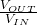:

•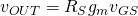•Thus, we have: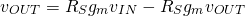Rearranging terms, we obtain:Just like our common collector amplifier, the common drain amplifier has unity voltage gainThe output voltage follows the input voltage: for this reason the common drain source amplifier is also called the source follower.

### Current Gain

Input is applied at the gate, but a JFET’s gate draws no current.  This circuit draws no current from the input (apart from the negligible amount passing through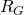), but supplies current at the output.  Current gain is infinite.

### Input ResistanceInput resistance is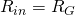and is very high.

### Output Resistance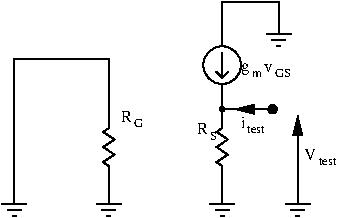To find the output resistance, we ground the input signal, and apply a test signal at the output.  Then, we calculate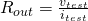:The input is grounded so, and our test signal is applied at the source terminal, so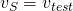: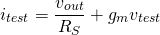We can now calculate our output resistance: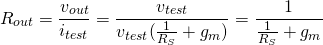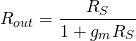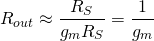The output resistance is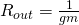which is low.

### Conclusion

Just like the common collector amplifier, the common source amplifier has:

• high input resistance :• low output resistance: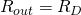• unity voltage gain
• current gain

And just like the common collector amplifier, this circuit makes an excellent buffer.  Its high input resistance prevents loading preceding stage, and its low output resistance allows to easily supply a certain voltage to the next stage.